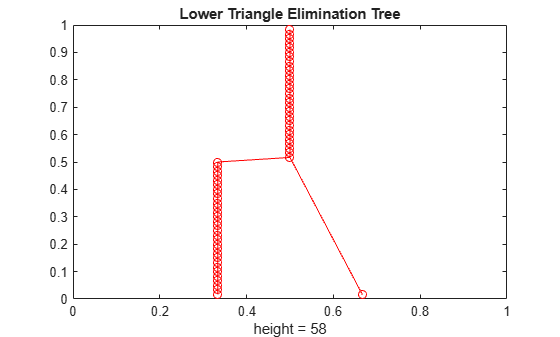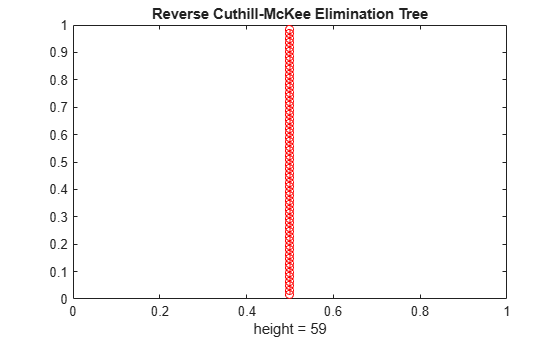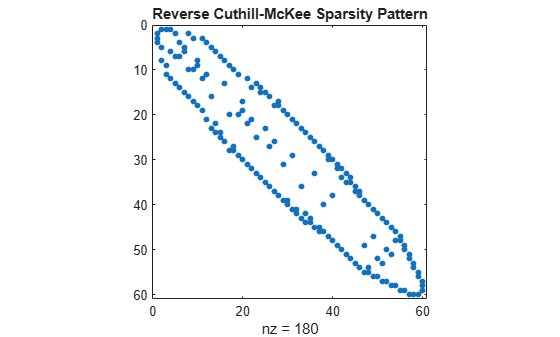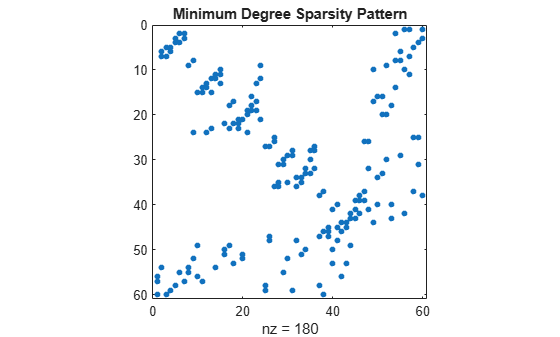# etree

Elimination tree

## Syntax

``p = etree(A)``
``p = etree(A,type)``
``[p,q] = etree(___)``

## Description

example

````p = etree(A)` returns an elimination tree of the square symmetric matrix whose upper triangle is that of `A`.```

example

````p = etree(A,type)` specifies the type of elimination tree. For example, if `type` is `"lo"`, then `etree` uses the lower triangle of `A` to construct the square symmetric matrix.```
````[p,q] = etree(___)` also returns the postorder permutation `q` of the elimination tree. You can specify two outputs with any of the input argument combinations in the previous syntaxes.```

## Examples

collapse all

Create a `7`-by-`7` tridiagonal matrix of zeros and ones.

`A = diag(ones(1,7)) + diag(ones(1,6),1) + diag(ones(1,6),-1)`
```A = 7×7 1 1 0 0 0 0 0 1 1 1 0 0 0 0 0 1 1 1 0 0 0 0 0 1 1 1 0 0 0 0 0 1 1 1 0 0 0 0 0 1 1 1 0 0 0 0 0 1 1 ```

Calculate an elimination tree of `A`. Each element `p(i)` of the elimination tree represents the parent of node `i`. `p(7)` is 0 because node 7 is the root of the tree.

`p = etree(A)`
```p = 1×7 2 3 4 5 6 7 0 ```

Create a `6`-by-`6` block diagonal matrix of zeros and ones.

```a = ones(3); b = zeros(3); B = [a b; b a]```
```B = 6×6 1 1 1 0 0 0 1 1 1 0 0 0 1 1 1 0 0 0 0 0 0 1 1 1 0 0 0 1 1 1 0 0 0 1 1 1 ```

Calculate an elimination tree of `B`. `etree` returns a forest with two elimination trees, indicated by the root nodes at indices 3 and 6.

`r = etree(B)`
```r = 1×6 2 3 0 5 6 0 ```

Calculate two different elimination trees of the `bucky` sparse matrix. The `"lo"` elimination tree uses the lower triangle of `A,` while the `"col"` elimination tree uses the matrix `A'*A`.

```A = bucky; p1 = etree(A,"lo"); p2 = etree(A,"col");```

Plot the elimination trees using `treeplot`.

```treeplot(p1) title("Lower Triangle Elimination Tree")``````treeplot(p2) title("Column Elimination Tree")```Calculate elimination trees of two permutations of the `bucky` sparse matrix. Use `symrcm` to create the symmetric reverse Cuthill-McKee ordering, and use `symamd` to create the symmetric approximate minimum degree ordering.

```A = bucky; r = symrcm(A); p1 = etree(A(r,r)); m = symamd(A); p2 = etree(A(m,m));```

Plot the elimination trees using `treeplot`. The reverse Cuthill-McKee elimination tree is a line of nodes, while the minimum degree elimination tree has multiple disjoint branches.

```treeplot(p1) title("Reverse Cuthill-McKee Elimination Tree")``````treeplot(p2) title("Minimum Degree Elimination Tree")```Plot the sparsity patterns using `spy`. The elimination tree of a matrix depends on its sparsity pattern, so the different sparsity patterns result in different elimination trees.

```spy(A(r,r)) title("Reverse Cuthill-McKee Sparsity Pattern")``````spy(A(m,m)) title("Minimum Degree Sparsity Pattern")```## Input Arguments

collapse all

Input matrix. `A` can be either full or sparse. If the elimination tree type is `"sym"` or `"lo"`, then `A` must be a square matrix. `etree` uses the sparsity structure of `A` to calculate the elimination tree.

Data Types: `double` | `logical`
Complex Number Support: Yes

Type of elimination tree, specified as `"sym"`, `"lo"`, `"col"`, or `"row"`. Use this argument to specify what matrix `etree` uses to compute the elimination tree.

• If `type` is `"sym"`, then `etree` constructs a square symmetric matrix using the upper triangle of `A` and returns the elimination tree of that matrix.

• If `type` is `"lo"`, then `etree` constructs a square symmetric matrix using the lower triangle of `A` and returns the elimination tree of that matrix.

• If `type` is `"col"`, then `etree` constructs the matrix `A'*A` and returns the elimination tree of that matrix, which is the column elimination tree of `A`.

• If `type` is `"row"`, then `etree` constructs the matrix `A*A'` and returns the elimination tree of that matrix, which is the row elimination tree of `A`.

## Output Arguments

collapse all

Elimination tree, returned as a numeric vector. `p(i)` represents the parent of node `i` in the tree, or `0` if `i` is a root.

Postorder permutation of elimination tree `p`, returned as a numeric vector.

You can use the postorder permutation of an elimination tree when computing the columns of a Cholesky factorization by hand. For a node `i` and its parent node `j` in an elimination tree `p`, column `i` must be completely computed before column `j` in the Cholesky factorization. The postorder permutation of `p` specifies an order in which to compute these columns that is consistent with this requirement. Use `chol` to compute the factorization directly.